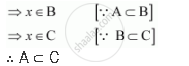CBSE (Arts) Class 11CBSE
Share

# Determine Whether the Statement is True Or False. If It is True, Prove It. If It is False, Give an Example. If a Subset B and B Subset C, Then a Subset C - CBSE (Arts) Class 11 - Mathematics

#### Question

determine whether the statement is true or false. If it is true, prove it. If it is false, give an example.

If A ⊂ B and B ⊂ C, then A ⊂ C

#### Solution

True

Let A ⊂ B and B ⊂ C.

Let x ∈ AIs there an error in this question or solution?

#### Video TutorialsVIEW ALL 

Solution Determine Whether the Statement is True Or False. If It is True, Prove It. If It is False, Give an Example. If a Subset B and B Subset C, Then a Subset C Concept: Subsets.
S